Next: The Manin constant Up: Invariants of Modular Abelian Previous: Mordell-Weil Groups and Torsion   Contents

# Analytic invariants

Definition 8.5.1 (Modular degree)   If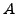is an optimal quotient of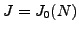the modular degree ofis the degree of the composite map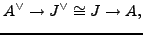where we may identify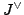with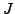sinceis a Jacobian of a curve with a rational point.

The period lattice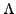forcan be described in terms of a pair of matrices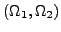such that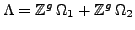. The volume of this lattice is one of the invariants which enters into the BSD Conjectures.

An analytic approach is the only known general way to compute the modular degree of an optimal quotientof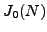. More precisely, there is a purely algebraic algorithm (which involves the theory of the analytic period lattice), which allows one to compute the modular degree. See [KS00] and the MAGMA source code. Whenhas dimension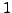there is an alternate algorithm due to Mark Watkins to compute the modular degree. It involves making computation of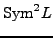explicit and using Flach's theorem.

Remark 8.5.2 (From Mark Watkins:)

"Flach's Theorem" should be (maybe) "Shimura's formula" or something. Flach's theorem relates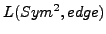to the Bloch-Kato conjecture, whereas the Shimura work relates it (via Rankin convolution) to the modular degree (at least for curves that are not semistable, getting the fudge factors correct probably is mentioned first in Flach, but he doesn't exactly work out the factors explicitly).

However, I think the best reference for the passage from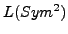to the modular degree is in Flach's paper:

   [ ]  MR1300880 (95h:11053) Flach, Matthias On the degree of
modular parametrizations. Seminaire de Theorie des Nombres, Paris,
1991--92, 23--36, Progr. Math., 116, Birkhaeuser Boston, Boston, MA,
1993. (Reviewer: Henri Darmon) 11G05 (11F30 11F33 11G40)


Problem 8.5.3   Is there any analogue of Watkins algorithm for any abelian varieties of dimension bigger than?

Remark 8.5.4 (From Mark Watkins)   When I visited Barcelona I talked with Jordi Quer about a variation for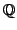-curves of Problem 8.5.3, but nothing ever happened with it.Next: The Manin constant Up: Invariants of Modular Abelian Previous: Mordell-Weil Groups and Torsion   Contents
William Stein 2006-10-20